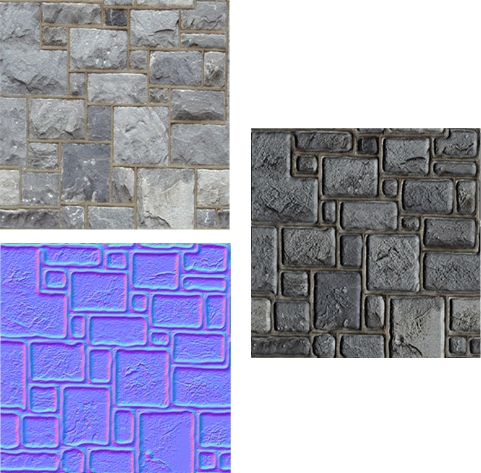# CUDA

CUDA, Compute Unified Device Architecture, is a parallel computing platform designed by NVIDIA. NVIDIA manufactures graphics cards that accelerate the layout of graphics to a display. In 1999, NVIDIA presented its GeForce 256 as “the world’s first ‘GPU’, or Graphics Processing Unit”. A GPU is an specialized processor designed to speed up 3D rendering. 3D rendering algorithms involve simple calculations, such the amount of light that each pixel receives for each frame, executed over and over, extremely quickly. Therefore, the GPU has been specialized to calculate simple math operations (i.e. matrix product) using lots of threads (parallel process unit).

Nowadays, developers can use this powerful architecture to operate on our own data using CUDA. NVIDIA provides the tools that developers need to take advantage of this technology.

## Bump mapping

One of the common algorithms used in 3D rendering is bump mapping. It takes a texture and changes the illumination behavior for each pixel in the mesh using the normal mapping coded for in another image.

The following code is for a fragment shader. This code renders the correct color of every pixel using, for each pixel of the image: the color of the texture (diffuse color), the normal of the surface in that point, and the light position.

``````uniform sampler2D sampler2d0;
uniform sampler2D sampler2d1;
```
```void main()
{
vec2 coordTex = (gl_Vertex.xy + 1.0) / 2.0;
```
```   vec4 color = texture2D(sampler2d0, coordTex);
vec4 normalMap = texture2D(sampler2d1, coordTex);
```
```   vec4 eyeVertex = gl_ModelViewMatrix * gl_Vertex;
```
```   vec3 normal = gl_NormalMatrix * normalMap.xyz;
normal = normalize(2*(normal-0.5));
```
```   vec3 lightDir = gl_LightSource.position.xyz - eyeVertex.xyz;
lightDir = normalize(lightDir);
```
```   float diffuse = dot(lightDir, normal);
```
```   gl_Position = gl_ProjectionMatrix * eyeVertex;
gl_FrontColor.rgb = diffuse * color.rgb;
gl_FrontColor.a = 1.0;
}
``````

It is not necessary to understand the code for this post. It is only important is to realize that the code operates with vectors and matrices and is extremely fast.Top-left: diffuse color, Bottom-left: normal mapping (rgb as n=(x,y,z)), right: result.

## SIS example

I wanted to test if CUDA can improve our daily work. To do that, I wrote a simple SIS epidemic simulation in Python and CUDA (using C++).

An SIS epidemic simulation runs on a network and simulates the behaviour between nodes, where infections can spread across edges. Each node is infected or susceptible. The infected infect their susceptible neighbors at each time-step with a defined probability, and can become susceptible again with another defined probability. These kind of simulations run until the network stabilizes, that is, when the percentage of infected nodes reaches a stable value.

Using a Barabási-Albert model graph (the first column of the results shows how many nodes and the link number for each step), the results are as follows:

 Graph creation Simulation Python CUDA Python CUDA 150000/10 3.11 1.51 36.9 2.27 300000/10 7.67 3.45 80.0 4.25 450000/10 11.20 5.67 126.0 6.13 600000/10 12.60 7.90 186.0 8.10

in seconds (SIS parameters: beta=0.012, mu=0.12)

## Pros/Cons

Working deeply with the results, I realized that there are some aspects that we need to take care before move all of our code to CUDA.

Pros:

• CUDA runs fast.
• With CUDA, we’ll use hardware we currently have but aren’t taking advantage of.
• We can create commands to run algorithms on a Unix environment and then run them from Python without taking care about CUDA stuff.

Cons:

• CUDA is harder to program than Python.
• It’s fast to test new algorithms in Python.
• CUDA is not available in our cluster
• The graphical interface in Ubuntu configures a timeout in the GPU to improve the user experience. In that case, our CUDA program can be interrupted if the timeout raised.

So, if you need to run a lot of simulations changing the parameters, probably it is better to run all of them in a cluster using the Python code. CUDA is good, but all depends on your project.